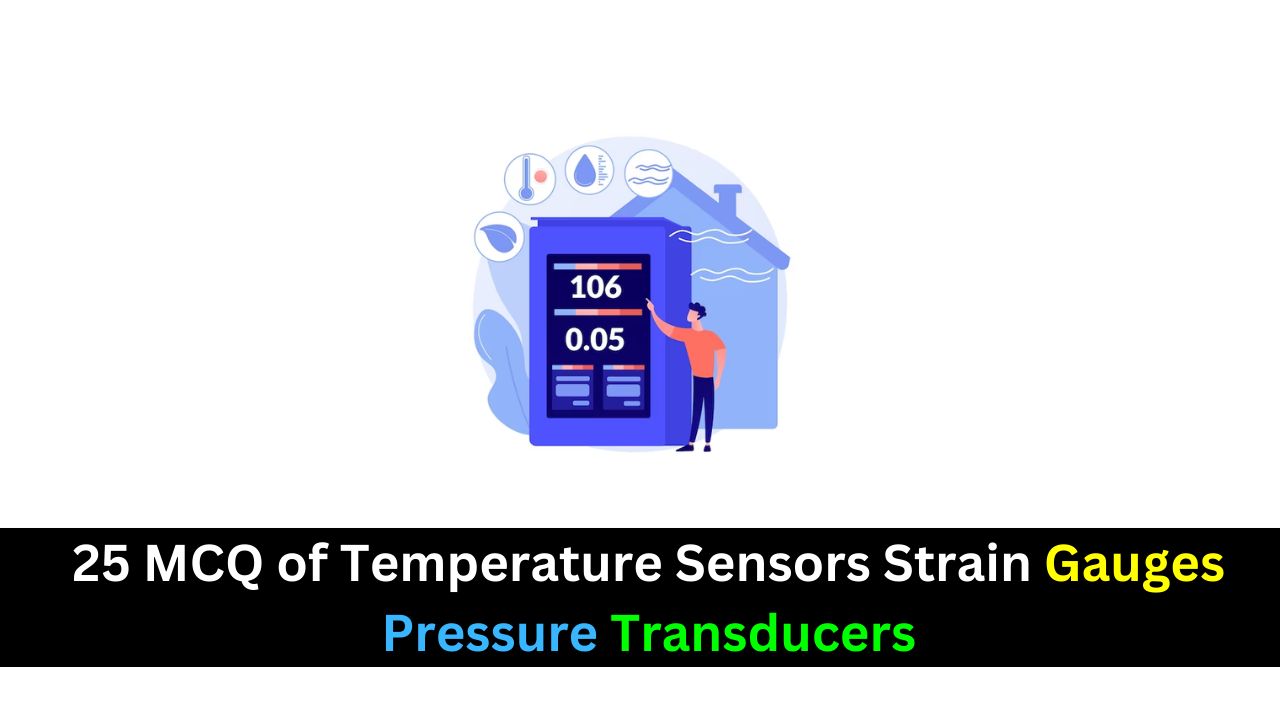Thursday, October 26

## 25 MCQ of Temperature Sensors Strain Gauges Pressure Transducers### A thermocouple

(a) produces a change in resistance for a change in temperature
(b) produces a change in voltage for a change in temperature
(c) is made of two dissimilar metals
Answer: (b) produces a change in voltage for a change in temperature

### In a thermocouple circuit, where each of the thermocouple wires is connected to a copper circuit board terminal,

(a) an unwanted thermocouple is produced (b) compensation is required
(c) a reference thermocouple must be used (d) answers (a), (b), and (c)

### A thermocouple signal conditioner is designed to provide

(a) gain (b) compensation (c) isolation
(d) common-mode rejection (e) all of the answers

### An RTD

(a) produces a change in resistance for a change in temperature
(b) has a negative temperature coefficient
(c) has a wider temperature range than a thermocouple
(d) all of these
Answer: (a) produces a change in resistance for a change in temperature

### The purpose of a 3-wire bridge is to eliminate

(a) nonlinearity of an RTD (b) the effects of wire resistance in an RTD circuit
(c) noise from the RTD resistance (d) none of these
Answer: (b) the effects of wire resistance in an RTD circuit

### A thermistor

(a) is less sensitive than an RTD
(b) can measure a greater temperature range than a thermocouple
(c) can be used in a constant-current configuration or a Wheatstone bridge
(d) all of the above
Answer: (c) can be used in a constant-current configuration or a Wheatstone bridge

### Both RTDs and thermistors are used in

(a) circuits that measure resistance (b) circuits that measure temperature
(c) bridge circuits (d) constant-current-driven circuits
(e) answers (b), (c), and (d) (f) answers (b) and (c) only

### When the length of a strain gauge increases,

(a) it produces more voltage (b) its resistance increases
(c) its resistance decreases (d) it produces an open circuit
Answer: (a) it produces more voltage

### A higher gauge factor indicates that the strain gauge is

(a) less sensitive to a change in length (b) more sensitive to a change in length
(c) has more total resistance (d) made of a physically larger conductor
Answer: (b) more sensitive to a change in length

### Many types of pressure transducers are made with

(a) thermistors (b) RTDs
(c) strain gauges (d) none of these

### Gauge pressure is measured relative to

(a) ambient pressure (b) a vacuum (c) a reference pressure

### The flow rate of a liquid can be measured

(a) with a string (b) with a temperature sensor
(c) with an absolute pressure transducer (d) with a differential pressure transducer
Answer: (d) with a differential pressure transducer

### A basic sample-and-hold circuit contains

(a) an analog switch and an amplifier
(b) an analog switch, a capacitor, and an amplifier
(c) an analog multiplexer and a capacitor
(d) an analog switch, a capacitor, and input and output amplifiers
Answer: (b) an analog switch, a capacitor, and an amplifier

### In a sample/track-and-hold circuit,

(a) the voltage at the end of the sample interval is held
(b) the voltage at the beginning of the sample interval is held
(c) the average voltage during the sample interval is held
(d) the output follows the input during the sample interval

### In an analog switch, the aperture time is the time it takes for the switch to

(a) fully open after the control switches from hold to sample
(b) fully close after the control switches from sample to hold
(c) fully open after the control switches from sample to hold
(d) fully close after the control switches from hold to sample
Answer: (b) fully close after the control switches from sample to hold

### An analog signal must be sampled at a minimum rate greater than

(a) twice the maximum frequency (b) twice the minimum frequency
(c) the maximum frequency (d) the minimum frequency
Answer: (a) twice the maximum frequency

### Quantization error in an ADC is due to

(a) poor resolution
(b) nonlinearity of the input
(c) a missing bit in the output
(d) a change in the input voltage during the conversion time
Answer: (c) a missing bit in the output

### Quantization error can be avoided by

(a) using a higher resolution ADC (b) using a sample-and-hold prior to the ADC
(c) shortening the conversion time (d) using a flash ADC

### Zero-voltage switching is commonly used in

(a) determining thermocouple voltage (b) SCR and triac power-control circuits
(c) balanced bridge circuits (d) RFI generation
Answer: (b) SCR and triac power-control circuits

### A major disadvantage of nonzero switching of power to a load is

(a) lack of efficiency (b) possible damage to the thyristor (c) RF noise generation